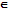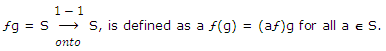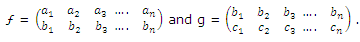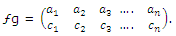# Describe the product of two permutations

Publish On: 2019-03-19

Total Post: 542

# Mjay Jollay

Total Post: 0

## ANS: Describe the product of two permutations

The product or composite of two permutations ƒ, gSn, denoted bythus the product of two permutations ƒ and g of degree n denoted by ƒg is obtained by first carrying out the operation defined by ƒ and then by g.

Let ƒ, gSn andHere the permutation g has been written in such a way that the first row of g coincides with the second row of ƒ. If the product of two permutations ƒ and g is denoted multiplicatively, i.e. by ƒg or ƒog then by definitionFor, ƒ replaces a1 by b1 and then g replaces b1 by c1 so that ƒg replaces a1 by c1. Similarly, ƒg replaces a2 by c2, a3 by c3, …., an by cn.

Obviously, ƒg is also a permutation of degree n. thus the product of two permutations of degree n is also a permutation of degree n.

Therefore, ƒ gSn, ∀ ƒ, gSn.